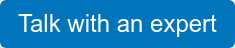OBJECTIVE

Track plants while machine is in motion to more precisely target where the plant is going to be instead of where it is seen.

APPROACH

Apply several equations of motion and time to the displacement, velocity, and acceleration of plants as the machinery / point of execution is what is in motion.

RESULTS

Well, it worked. But, there’s still quite a bit of fine tuning to be done here.

KEY LEARNINGS

Everything changes as the plant changes (grows) and it’s surface area changes in relativity to the pre-existing point of reference. Because… now the target is bigger. This means it’s relativity to the placement of the camera is closer, as well as the point fo execution (sprayer nozzle).

If farm machinery is moving, and our target (the plant), is passing us by, and our execution hardware, (nozzle), if X number fo feet off the ground, then we need to calculate for all of that. We need the system to be sending application where the plant is going to be, not where it is. This means we need to factor in the math behind motion and time, (understanding distance can be measured in time). See below more info on coming to this conclusion.

Terms Related to Motion

Position: It is defined as the location of the particle with respect to the chosen reference point. It is denoted by 𝑟⃗ r→.

Displacement: It is defined as the change in position of the particle with respect to the reference point. We calculate it by taking the difference between the final and the initial position. It is denoted by 𝑆⃗ S→.

Distance: It is defined as the total length of the path taken by the particle.

Velocity: The rate of change of displacement with respect to time is called velocity. It is denoted by 𝑣⃗ v→ or 𝑢⃗ u→.

Speed: The rate of change of distance with respect to time is known as speed.

Acceleration: The rate of change of velocity with respect to time is called acceleration. It is denoted by 𝑎⃗ a→.

Negative acceleration is also sometimes referred to as retardation.

Displacement, velocity, and acceleration are vector quantity while speed and distance are scalar quantities.

We also defined the average and instantaneous values of these parameters.

Instantaneous values are measure at a particular instance, while the average values are considered over a period of time.

Example: The average velocity of a body moving in a circular path in one revolution will be zero as the initial and final positions are the same. Still, the instantaneous velocity of that particle may or may not be zero.

Equations of Motion

Initial velocity is denoted by 𝑢⃗ u→.

The final velocity is denoted by 𝑣⃗ v→.

Displacement is denoted by 𝑠⃗ s→.

Acceleration is denoted by 𝑠⃗ s→.

It is important to note that acceleration is considered to be constant. If the acceleration is not constant, the equation of motions will not hold true.

The first equation of motion gives the relation between the initial velocity, final velocity, acceleration, and the time interval. It is given as,

𝑣⃗ =𝑢⃗ +𝑎⃗ 𝑡v→=u→+a→t

The second equation of motion gives the relation between the displacement, initial velocity, acceleration, and the time interval. It is given as,

𝑠⃗ =𝑢⃗ 𝑡+12𝑎⃗ 𝑡2s→=u→t+12a→t2

The third equation of motion gives us the relation between the final and the initial velocities, acceleration, and displacement. It is given as,

𝑣2–𝑢2=2𝑎→⋅𝑠→

Example on Motion and Time

A particle moves in a circle with a diameter of 14m14m with some constant speed and completes the circular path in 2020 seconds. Find the average velocity and average speed from 𝑡=0t=0 to 𝑡=10sect=10sec
Given,
The diameter of the circular path is 14m14m.
Time taken to complete one revolution is 2020 seconds.
The average speed is given by the total distance divided by the total time taken.
speed=distancetimetakenspeed=distancetimetaken
speed=2𝜋𝑟20–0speed=2πr20–0
𝑣=2𝜋𝑟20–0v=2πr20–0
⇒𝑣=2×227×720–0=2.2ms–1⇒v=2×227×720–0=2.2ms–1
Since it is given in the question that the speed is constant, therefore, in any time interval, the average speed will be equal to the average speed.
Average velocity will be given by total displacement divided by the total time taken,
In 2222 seconds, the particle completes one revolution, so in 1111 seconds, it will complete half the revolution; therefore, the displacement will be equal to the diameter.
Thus, the velocity will be,
𝑣=𝑑𝑡v=dt
⇒𝑣=1410=1.4ms–1⇒v=1410=1.4ms–1

A particle is moving with retardation of 5m5m. The initial velocity of the particle is 20m/s20m/s, then find the distance travelled before it comes to rest.
Given,
The initial velocity of the particle is 20m/s20m/s.
The acceleration of the particle is –5ms–2–5ms–2.
Since the particle comes to rest, the final velocity will be zero.
From the third equation of motion we have,
𝑣2–𝑢2=2𝑎𝑠v2–u2=2as
Putting in the values, we get,
02–202=2(–5)𝑠02–202=2(–5)s
⇒𝑠=40m

credit: vishnus_c - emibe.com